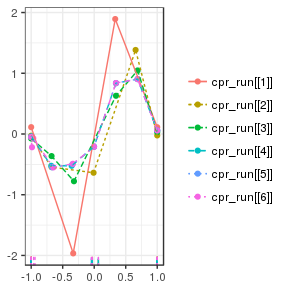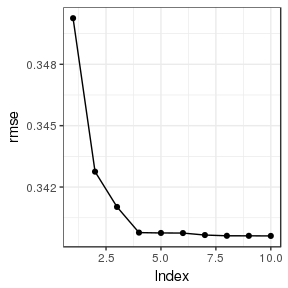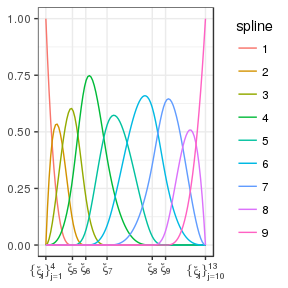# The Control Polygon Reduction Package

#### 2017-03-06

The cpr package provides several tools for finding parsimonious B-spline regression models via control polygon reduction. This vignette is an overview of the tools provided by the package.

This vignette is not complete. The details will be filled in soon. As for now, this is a placeholder for many topics to come. A Journal of Statistical Software paper is under development. That paper will become the foundation for this vignette.

A brief overview of the Control Polygon Reduction and Control Net Reduction method for finding parsimonious B-spline regression models are presented first. The later sections of this vignette provide extended detail on the additional tools provided by the cpr package.

# Needed packages to run the examples in this vignette
library(cpr)
library(splines)

# CPR: Control Polygon Reduction

Coming Soon A full description of the CPR method, including the background on B-splines and assessing knot influence will be provided soon.

The quick overview: for a uni-variable B-spline regression model $\boldsymbol{y}= f(\boldsymbol{x}) + \boldsymbol{Z}_{f} \boldsymbol{\beta} + \boldsymbol{Z}_{r} \boldsymbol{b} + \epsilon$ where $$\boldsymbol{y}$$ is a function of one variable $$x$$ with other (optional) fixed effects $$\boldsymbol{Z}_{f} \beta$$ and (optional) random effects $$\boldsymbol{Z}_{r} \boldsymbol{b},$$ we are interested in modeling the function $$f\left( \boldsymbol{x} \right)$$ with B-splines.

The B-spline function is $f \left(\boldsymbol{x}\right) \approx \boldsymbol{B}_{k, \boldsymbol{\xi}} \left( \boldsymbol{x} \right) \boldsymbol{\theta}$ were $$\boldsymbol{B}$$ is the basis matrix defined by the polynomial order $$k$$ and knot sequence $$\boldsymbol{\xi},$$ and the regression coefficients $$\boldsymbol{\theta}.$$

The Control Polygon Reduction (CPR) approach to finding parsimonious regression models with a good quality of fit is to start with a high cardinal knot sequence, assess the relative influence of each knot on the spline function, remove the least influential knot, refit the regression model, reassess the relative influence of each knot, and so forth until all knots knots have been removed. The selection of a regression model is made by looking at the regression models of sequentially larger knot sequences until it is determined that the use of an additional knot does not provide any meaningful improvements to the regression model.

There are only four steps that the end user needs to take to use the CPR algorithm within the cpr package.

1. Construct a control polygon with a large cardinal knot sequence, using cp.
2. Run the CPR algorithm via a call to cpr.
3. Use diagnostic plots to determine the preferable model.
4. Save the preferable model.

Here is an example.

# Construct the initial control polygon
initial_cp <- cp(log10(pdg) ~ bsplines(day, df = 54), data = spdg)

# Run CPR
cpr_run    <- cpr(initial_cp)

There are two types of diagnostic plots, 1) sequential control polygons, and 2) root mean squared error (RMSE) by model index.

In the plot below, there is no major differences in the shape of the control polygon between model index 4, 5, and 6. As such, we would conclude that model index 4 is sufficient for modeling the data.

# sequential control polygons
plot(cpr_run, color = TRUE)Further, in the plot below, we see that there is very little improvement in the RMSE from model index 4 to 5, and beyond. As with the plot above, we conclude that model index 4 is sufficient for modeling the data.

# RMSE by model index
plot(cpr_run, type = 'rmse', to = 10)Extract the model. To save memory when running CPR, the default is to omit the regression model objects from the control polygons with in the cpr_run object. To get the regression model back, you can extract the knot locations and build the model yourself, or update the selected_cp object to retain the fit.

selected_cp <- cpr_run[]
selected_cp$iknots ##  -0.04429203 -0.02992955 0.06601307 selected_cp <- update(selected_cp, keep_fit = TRUE) selected_fit <- selected_cp$fit
coef_matrix <- summary(selected_fit)\$coef
dimnames(coef_matrix)[] <- paste("Vertex", 1:7)
coef_matrix
##             Estimate  Std. Error    t value      Pr(>|t|)
## Vertex 1 -0.04067426 0.009447938  -4.305094  1.675568e-05
## Vertex 2 -0.52353915 0.020364344 -25.708619 7.278010e-144
## Vertex 3 -0.52379368 0.018812577 -27.842740 5.154301e-168
## Vertex 4 -0.20824755 0.008756483 -23.782101 1.252151e-123
## Vertex 5  0.83530262 0.019968306  41.831421  0.000000e+00
## Vertex 6  0.90521730 0.019961035  45.349217  0.000000e+00
## Vertex 7  0.05860973 0.009570407   6.124058  9.259636e-10

If you wanted to run this analysis with a better modeling approach, e.g., using mixed effect models handle the multiple observations within a subject, you can specify the regression approach via the method argument in the cp call.

library(lme4)
initial_lmer_cp <- cp(log10(pdg) ~ bsplines(day, df = 54) + (1 | id),
data = spdg,
method = lmer)

# CNR: Control Net Reduction

Coming Soon Control Net reduction is the natural extension of CPR from uni-variable functions to multi-variable functions. Details on this work will be added to this vignette soon.

# Additional Tools

The CPR and CNR algorithms rely on B-splines, control polygons, tensor products of B-splines, and control nets. The following sections provide additional detail on the tools within the cpr package.

## B-Splines

Base R includes the splines package and the splines::bs call for building B-splines. The cpr package provides the cpr::bsplines call as there are certain default behaviors and additional required meta-data storage requirements needed for the CPR method that the splines::bs call does not provide. In this section we compare splines::bs and cpr::bsplines so that end users can translate between the two functions.

splines::bs cpr::bsplines
Arguments
x x
df df
knots iknots
degree = 3 order = 4L
Boundary.knots = range(x) bknots = range(x)
intercept = FALSE
Attributes
dim dim
degree order
knots iknots
Boundary.knots bknots
intercept
xi
xi_star
class class

A major difference between the two functions is related to the intercept argument of bs. By default, bs will omit the first column of the basis whereas bsplines will return the whole basis. The omission of the first column of the basis generated by bs allows for additive bs calls to be used on the right-hand-side of a regression formula and generate a full rank design matrix. If additive bsplines calls, or additive bs with intercept = TRUE, are on the right-hand-side of the regression equation the resulting design matrix will be rank deficient. This is a result of the B-splines being a partition of unity. As the CPR algorithm is based on having the whole basis, the bsplines function is provided to make it easy to work with the whole basis without having to remember to use non-default settings in bs.

Both functions use x, a numeric vector, as the first argument. The degrees of freedom, df, argument is slightly different between the two functions; the return object from bs depends on the values of df and intercept whereas bsplines always returns the full basis and thus df will be equal the number of columns of the returned basis.

bs uses the polynomial degree whereas bsplines uses the polynomial order (order = degree + 1) to define the splines. The default for both bs and bsplines is to generate cubic B-splines.

Specifying the internal knots and boundary knots are the same between the two functions save the name of the arguments. For both bs and bsplines only the degrees of freedom or the internal knots need to be specified. If the end user specifies both, the specified knots take precedence. If only the degrees of freedom are specified then bs will generate internal knots via a call equivalent to

stats::quantile(x, probs = seq(0, 1, length = length(knots) + 2L)[-c(1, length(knots) + 2L)].  

The default behavior for bsplines is nearly the same, only that a call to trimmed_quantile is made.

bmat <- bsplines(x = seq(0, 6, length = 500), iknots = c(1.0, 1.5, 2.3, 4.0, 4.5))
plot(bmat)# Topics to be written:

• Construction of control polygons, cp
• Tensor products, in general build_tensor and of B-splines btensor
• Construction of control nets, cn
• Assessing the relative influence of a knot
• Plotting splines and surfaces

# Session Info

print(sessionInfo(), local = FALSE)
## R version 3.3.2 (2016-10-31)
## Platform: x86_64-pc-linux-gnu (64-bit)
## Running under: Debian GNU/Linux 8 (jessie)
##
## attached base packages:
##  splines   stats     graphics  grDevices utils     datasets  methods
##  base
##
## other attached packages:
##  cpr_0.2.3    knitr_1.15.1
##
## loaded via a namespace (and not attached):
##   Rcpp_0.12.9      magrittr_1.5     MASS_7.3-45      munsell_0.4.3
##   colorspace_1.3-2 lattice_0.20-34  R6_2.2.0         minqa_1.2.4
##   plyr_1.8.4       stringr_1.2.0    dplyr_0.5.0      tools_3.3.2
##  grid_3.3.2       gtable_0.2.0     nlme_3.1-128     DBI_0.5-1
##  htmltools_0.3.5  lazyeval_0.2.0   yaml_2.1.14      lme4_1.1-12
##  rprojroot_1.2    digest_0.6.12    assertthat_0.1   tibble_1.2
##  Matrix_1.2-7.1   tidyr_0.6.1      ggplot2_2.2.1    nloptr_1.0.4
##  evaluate_0.10    rmarkdown_1.3    labeling_0.3     stringi_1.1.2
##  scales_0.4.1     backports_1.0.5`